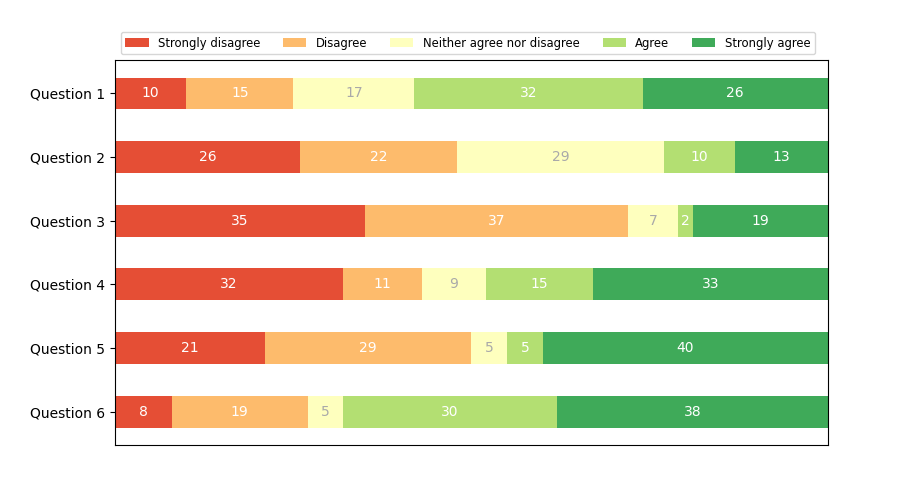# Discrete distribution as horizontal bar chart¶

Stacked bar charts can be used to visualize discrete distributions.

This example visualizes the result of a survey in which people could rate their agreement to questions on a five-element scale.

The horizontal stacking is achieved by calling barh() for each category and passing the starting point as the cumulative sum of the already drawn bars via the parameter left.

import numpy as np
import matplotlib.pyplot as plt

category_names = ['Strongly disagree', 'Disagree',
'Neither agree nor disagree', 'Agree', 'Strongly agree']
results = {
'Question 1': [10, 15, 17, 32, 26],
'Question 2': [26, 22, 29, 10, 13],
'Question 3': [35, 37, 7, 2, 19],
'Question 4': [32, 11, 9, 15, 33],
'Question 5': [21, 29, 5, 5, 40],
'Question 6': [8, 19, 5, 30, 38]
}

def survey(results, category_names):
"""
Parameters
----------
results : dict
A mapping from question labels to a list of answers per category.
It is assumed all lists contain the same number of entries and that
it matches the length of *category_names*.
category_names : list of str
The category labels.
"""
labels = list(results.keys())
data = np.array(list(results.values()))
data_cum = data.cumsum(axis=1)
category_colors = plt.colormaps['RdYlGn'](
np.linspace(0.15, 0.85, data.shape))

fig, ax = plt.subplots(figsize=(9.2, 5))
ax.invert_yaxis()
ax.xaxis.set_visible(False)
ax.set_xlim(0, np.sum(data, axis=1).max())

for i, (colname, color) in enumerate(zip(category_names, category_colors)):
widths = data[:, i]
starts = data_cum[:, i] - widths
rects = ax.barh(labels, widths, left=starts, height=0.5,
label=colname, color=color)

r, g, b, _ = color
text_color = 'white' if r * g * b < 0.5 else 'darkgrey'
ax.bar_label(rects, label_type='center', color=text_color)
ax.legend(ncol=len(category_names), bbox_to_anchor=(0, 1),
loc='lower left', fontsize='small')

return fig, ax

survey(results, category_names)
plt.show()References

The use of the following functions, methods, classes and modules is shown in this example:

Keywords: matplotlib code example, codex, python plot, pyplot Gallery generated by Sphinx-Gallery